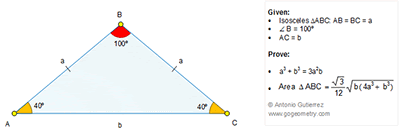## Sunday, November 13, 2016

### Geometry Problem 1287 Isosceles Triangle, 40-100-40 Degrees, Congruence, Area, Metric Relations, Measurement

Geometry Problem. Post your solution in the comment box below.
Level: Mathematics Education, High School, Honors Geometry, College.

This entry contributed by Sumith Peiris, Moratuwa, Sri Lanka.

Click on the figure below to view more details of problem 1287.1.Problem 1287
In extension of the CB passes point D such that AD=AC=b.Then the triangles ABC and CAD are similar.So a/b=b/CD or CD=b^2/a,BD=CD-CB=b^2/a-a. But <BAD=100-40=60,then BD^2=
a^2+b^2-ab =(b^2/a-a)^2 so a^3+b^3=3a^2b.
Draw BK perpendicular in AC then BK^2=a^2-b^2/4.
Let area triangle ABC is (ABC)=(AC.BK)/2 then substituting the BKand using a^3+b^3=3a^2b we have the effect.
APOSTOLIS MANOLOUDIS 4 HIGH SCHOOL KORYDALLOS PIRAEUS GREECE

2.From A, draw a line at 20 degrees to AC and length = a and form an equilateral triangle ABD.
Let BD intersect AC at E.
If you observe the triangle BEC is similar to ABC
Hence BE=EC=a^2/b
Therefore AE=b-a^2/b=b^2-a^2/b
=> AE^2=(b^2-a^2/b)^2 --------------(1)
Now consider the triangle ABE where m(ABE)=60
AE^2=AB^2+BE^2-2.AB.BE.COS60
=> AE^2=a^2+a^4/b^2-a^3/b---------------(2)

From (1) & (2)
(b^2-a^2/b)^2=a^2+a^4/b^2-a^3/b
=> a^3+b^3=3a^2b

3.Let area triangle ABC is (ABC) then (ABC)^2=(b^2.BK^2)/4=b^2(4a^2-b^2)/16. And (ABC)^2=3/144.b(4a^3+b^3).Let b^2(4a^2-b^2)/16=3/144.b(4a^3+b^3) or
(4a^3+b^3)/3=b(4a^2-b^2) or a^3+b^3=3a^2b which applies.
APOSTOLIS MANOLOUDIS 4 HIGH SCHOOL KORYDALLOS PIRAEUS GREECE